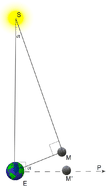# 21.1: Does the Earth Revolve Around the Sun?

•• Contributed by CK12
• CK12

Aristarchus of Samos, an early Greek astronomer (about 310 to 230 BC), was the first to suggest that the Earth revolved around the Sun, rather than the other way around. He gave the first estimate of the distance of the Moon (See the chapter “Estimating the Distance to the Moon"), and it was his careful observation of a lunar eclipse -- pin-pointing the Sun's position on the opposite side of the sky--that enabled Hipparchus, 169 years later, to deduce the precession of the equinoxes (See the chapter “Precession").

Except for one calculation -- an estimate of the distance and size of the Sun -- none of Aristarchus's work has survived. However, one could guess why he believed that the Sun, not the Earth, was the central body around which the other one revolved. His calculation suggested that the Sun was much bigger than the Earth -- a watermelon, compared to a peach -- and it seemed unlikely that the larger body would orbit one so much smaller.

Here we will develop a line of reasoning somewhat like the one Aristarchus used (for his actual calculation, see reference at the end). Aristarchus started from an observation of a lunar eclipse (See the chapter “Estimating the Distance to the Moon"). At such a time the Moon moves through the Earth's shadow, and what Aristarchus saw convinced him that the shadow was about twice as wide as the Moon. Suppose the width of the shadow was also the width of the Earth (actually it is less -- see below, also here). Then the diameter of the Moon would be half the Earth's.Figure $$\PageIndex{1}$$: Illustration of Aristarchus' calculation.

Aristarchus next tried to observe when exactly half the moon was sunlit. For this to happen, the angle Earth-Moon-Sun ($$\angle EMS$$ in the drawing here) must be exactly 90 degrees.

Knowing the Sun's motion across the sky, Aristarchus could also locate the point $$P$$ in the sky, on the Moon's orbit (near the ecliptic), which was exactly 90 degrees from the direction of the Sun as seen from Earth. If the Sun were very, very far away, the half-moon would also be on this line, at a position like M' (drawn with a different distance scale, for clarity).

Aristarchus estimated, however, that the direction to the half-Moon made a small angle a with the direction to $$P$$, about 1/30 of a right angle or 3 degrees.

As the drawing shows, $$\angle EMS$$ (Earth-Moon-Sun) then also must equal 3 degrees. If $$R_s$$ is the Sun's distance and $$R_m$$ the Moon's, a full 360 degree circle around the Sun at the Earth's distance has a length of $$2 \pi R_s$$. The distance $$R_m\,=\,EM$$ is then about as long as an arc of that circle covering 3 degrees, or 1/120 of the full circle. It follows that: $R_m\,=\,\frac{2\pi R_s}{120}\approx \frac{R_s}{19}$ Therefore, $frac{R_s}{R_m}\approx 19$, making the Sun -- according to Aristarchus -- 19 times more distant than the Moon. Since the two have very nearly the same size in the sky --- even though one of them is 19 times more distant, the Sun must also be 19 times larger in diameter than the Moon.

If the Moon's diameter is half the size of the Earth's, the Sun must be 19/2 or nearly 10 times wider than the Earth. The effect described in the figures of the next section modifies this argument somewhat (http://www.phy6.org/stargaze/Sshadow.htm), making the Earth 3 times wider than the Moon, not twice. If Aristarchus had observed correctly, that would make the Sun's diameter 19/3 times --- a bit more than 6 times --- than the Earth.

Actually, he had not! His method does not really work, because in actuality the position of the half-Moon is very close to the line OP. The angle $$\alpha$$, far from being 3 degrees, is actually so small that Aristarchus could never have measured it, especially without a telescope. The actual distance to the Sun is about 400 times that of the Moon, not 19 times, and the Sun's diameter is similarly about 400 times the Moon's and more than 100 times the Earth's.

But it makes no difference. The main conclusion, that the Sun is vastly bigger than Earth, still holds. Aristarchus could just as well have said that the angle $$\alpha$$ was at most 3 degrees, in which case the Sun was at least 19 times more distant than the Moon, and its size at least 19/3 times that of Earth. In fact he did say so -- but he also claimed it was less than 43/6 times larger than the Earth (Greeks used simple fractions -- they knew nothing about decimals), which was widely off the mark. But it makes no difference -- as long as the Sun is much bigger than the Earth, it makes more sense that it, rather than the Earth, is at the center.

Good logic, but few accepted it, not even Hipparchus or Ptolemy. In fact, the opposite argument was made: if the Earth orbited the Sun, it would be on opposite sides of the Sun every 6 months. If that distance was as big as Aristarchus claimed it to be, would not the positions of the stars differ when viewed from two spots so far apart? We now know the answer: the stars are so far from us, that even with the two points 20 times further apart than Aristarchus had claimed, the best telescopes can barely observe the shift of the stars. It took nearly 18 centuries before the ideas of Aristarchus were revived by Copernicus.